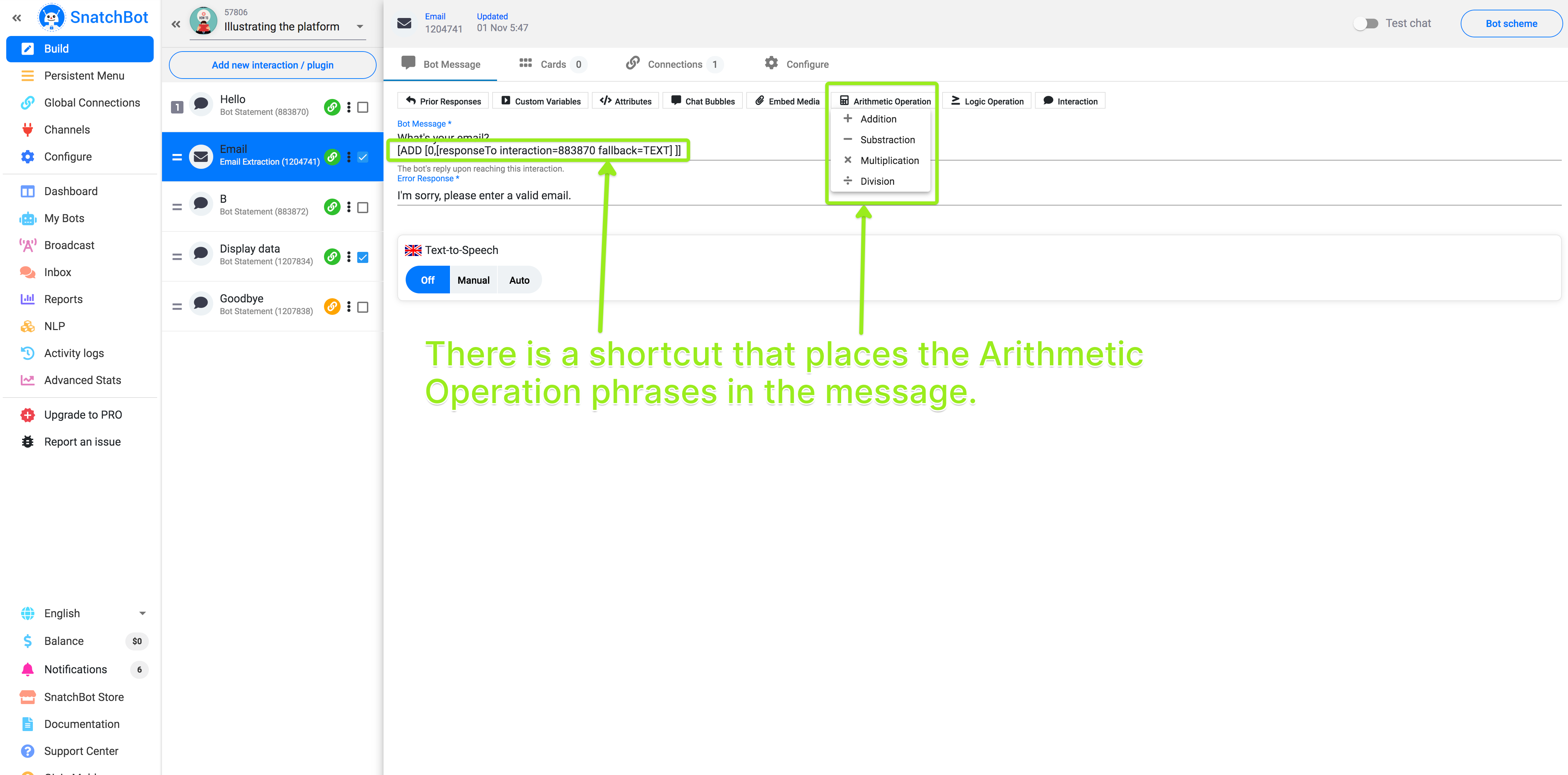# Arithmetical functions

Addition, subtraction, multiplication, and division are four arithmetical functions that the bot can perform.

If you are designing a chatbot that deals with payments, keeping score or any kind of numerical activity, you’ll want the chatbot to be able to perform arithmetical functions.

There are four arithmetical functions you can perform, listed below with the form that the function must take.

Arithmetical functions

[ADD [x, y, z, etc.]]

Subtraction:

[SUB [x, y, z, etc.]]

Multiplication:

[MUL [x, y, z, etc.]]

Division:

[DIV [x, y, z, etc.]]

Each of these works the same way, performing actions on the numbers inside the inner square brackets. The numbers are separated from each other by commas and are read from left to right. Numbers can be whole or decimal (decimal numbers are read up to the second place, e.g. 0.01 but not 0.014, note: decimal separators must be points, not commas).

### 📘Examples

[ADD [1, 3, 7]] = 1 + 3 + 7 = 11
[SUB [1, 3, 7]] = 1 - 3 - 7 = -9
[MUL [1, 3, 7]] = 1 3 7 = 21
[DIV [1, 3, 7]] = 1 / 3 / 7 = 0.05

The result is always rounded to a number with two figures after decimal point (so, 1 divided by 3, divided by 7 is 0.047619… and results in the rounded figure of 0.05)

You can find a shortcut to these functions above the message box of an interaction.# Using Custom Variables in Arithmetical Operations

Custom Variables whose value type is a number can be used instead of inserting a number directly.

Example 1

Interaction 1: Hi! Let’s divide three numbers. Please pick your first number.
Interaction 2: Great! Pick a second one. [customVar A=[responseTo interaction=1 fallback=TEXT]]
Interaction 3: Excellent, and now the third one. [customVar B=[responseTo interaction=2 fallback=TEXT]]
Interaction 4: The result is [DIV [[showVar=A], [showVar=B], [responseTo interaction=3 fallback=TEXT]]].

More complex constructions

Suppose you have already defined a Custom Variable A as in Example 1 and the bot has asked a numerical question at Interaction 37458.

[showVar=[extractedData interaction=37458 fallback=TEXT]]
[customVar=[responseTo interaction=37458 fallback=TEXT]]

Then you can combine your data in various arithmetical calculations such as:

[ADD [1, [showVar=A], [responseTo interaction=46541]]

This will create a value of 1+ the user’s choice in regard to Custom Variable A and the user’s response to Interaction 46541.

## Watch this video tutorial to learn more about "Carrying out arithmetic with your chatbot on the SnatchBot platform"

Next Steps

How you can carry out Logic Operations with your chatbot.